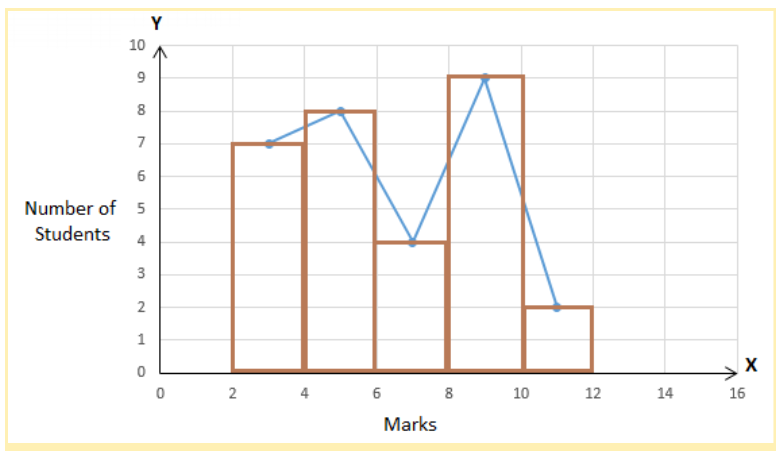# RBSE Maths Class 9 Chapter 15: Important Questions and Solutions

RBSE Maths Chapter 15 – Statistics Class 9 Important questions and solutions are given here. The important questions and solutions of Chapter 15, available at BYJU’S, have detailed steps along with the graphs. Also, RBSE Class 9 solutions are provided at BYJU’S improving their knowledge on all the concepts. It also enhance their analytical and logical skills.

Chapter 15 of the RBSE Class 9 Maths starts with the introduction of Statistics with the definition of primary and secondary data, along with the methods of collection. Four exercises exist in the chapter that contains questions on drawing histograms, frequency polygons and combination of both. Also, students can learn how to find the mean, median and mode of ungrouped data using simple formulas.

## RBSE Maths Chapter 15: Exercise 15.1 Textbook Important Questions and Solutions

Question 1: What are primary and secondary data? Clarify the difference.

Solution:

Data which is collected for the first time by an investigator for a specific purpose is called primary data.

Data which is not originally collected rather obtained in published or unpublished form is called secondary data.

Difference between primary and secondary data:

 Primary Data Secondary Data Primary data is original data and it is collected through statistical methods Secondary data can be obtained in the form of published or unpublished source Data collected by the statistical investigator himself Data collected by some other person for some other purpose This data collected for a specific purpose Data available in the form of records, reports and so on.

Question 2: Describe the methods of collection of primary data.

Solution:

(i) Direct personal investigation

In this method, the investigator directly contacts the persons from whom he is required to collect the information.

(ii) Indirect investigation

If the field of investigation is large/wide, then the investigator chooses one of the following methods to get the required information.

• Schedules to be filled in by enumeration
• Information through questions are to be filled in by informants
• Information through local sources or correspondents
• Indirect investigation through experts

## RBSE Maths Chapter 15: Exercise 15.2 Textbook Important Questions and Solutions

Question 3: Given below are the weights (in kg) of 30 students of class X of a school:

34, 34, 36, 37, 38, 33, 34, 35, 36, 37, 38, 33, 34, 35, 34, 33, 37, 35, 34, 36, 38, 36, 35, 34, 35, 37, 38, 34, 35, 35.

Prepare a frequency distribution table for the above data.

Solution:

The frequency distribution table for the given data is: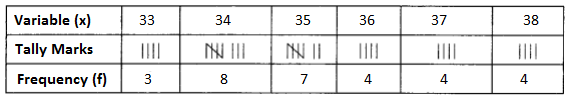Total = 3 + 8 + 7 + 4 + 4 + 4 = 30

Question 4: Three coins were tossed 30 times. Each time the number of tails occuring was noted down as follows.

1 3 1 1 2 2 0 1 2 1

2 3 1 3 0 0 1 2 2 1

3 0 1 1 2 3 3 2 2 0

Prepare a frequency distribution table for the data given above.

Solution:

The frequency distribution table for the given data is: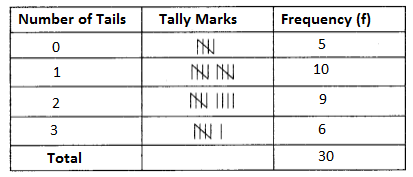Question 5: The blood groups of 30 students of class X are recorded as follows:

AB O O A B O B O B A O B A O O

B AB O B A O O AB B A O B A B O

Present this data in the form of a frequency distribution table. Find out which is the most common and which is the rarest blood group among these students.

Solution:

The frequency distribution table for the given data is: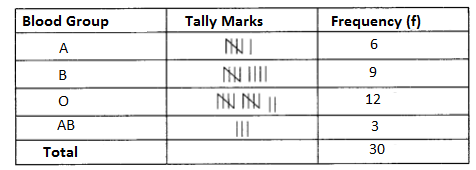Question 6: Following are the marks obtained by 30 students of class IX in an examination. Use these marks to prepare a frequency table of 5 classes of class size 10.

19 27 40 3 33 41 18 8 20 0 23 49 16 36 14

39 6 12 29 28 22 24 37 10 23 38 35 9 49 23

Solution:

From the given data,

Maximum marks = 49

Minimum marks = 0

Range = Maximum marks – Minimum marks = 49 – 0 = 49

Class size or class interval = 10 (given)

Number of intervals = 49/10 = 4.9 (taking as 5)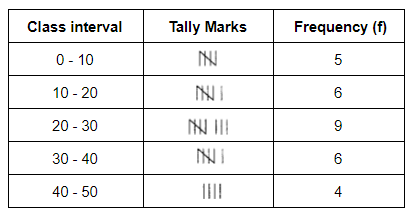Total frequency = 5 + 6 + 9 + 6 + 4 = 30

Question 7: Prepare a frequency distribution table for the following distribution of taking class interval 5.

13 11 8 19 0 44 27 10 8 35

13 27 30 17 43 23 19 43 17 7

Solution:

From the given data,

Minimum value = 0

Maximum value = 44

Range = 44 – 0 = 44

Class interval = 5 (given)

Number of classes = 44/5 = 8.8 (taking as 8)

 Class interval Tally Marks Frequency (f) 0 – 5 | 1 5 – 10 ||| 3 10 – 15 |||| 4 15 – 20 |||| 4 20 – 25 | 1 25 – 30 || 2 30 – 35 | 1 35 – 40 | 1 40 – 45 ||| 3

Total frequency = 1 + 3 + 4 + 4 + 1 + 2 + 1 + 1 + 3 = 20

## RBSE Maths Chapter 15: Exercise 15.3 Textbook Important Questions and Solutions

Question 8: From the following table draw a histogram.

 Class 0 – 20 20 – 40 40 – 60 60 – 80 80 – 100 Frequency 5 6 12 4 3

Solution:

Take class intervals on the X-axis and frequency values on the Y-axis with suitable scale.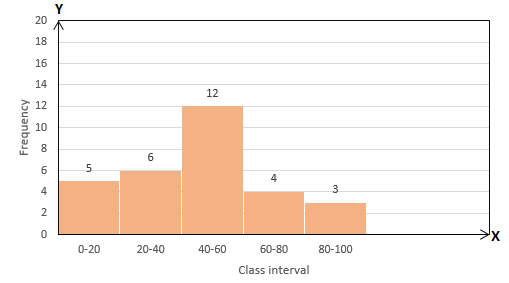Question 9: From the following table draw a histogram.

 Class 3 – 6 6 – 12 12- 13 13 – 14 14 – 15 Frequency 150 420 100 110 50

Solution:

Take the class interval along x-axis and the frequency on the y-axis. Since, the intervals are not of equal width, we have to construct the rectangles with the classes as their bases and their corresponding heights will be calculated as follows:

Height = Frequency/Size of the corresponding class

For 3 – 6: 150/3 = 50

For 6 – 12: 420/6 = 70

For 12 – 13: 100/1 = 100

For 13 – 14: 110/1 = 110

For 14 – 15: 50/1 = 50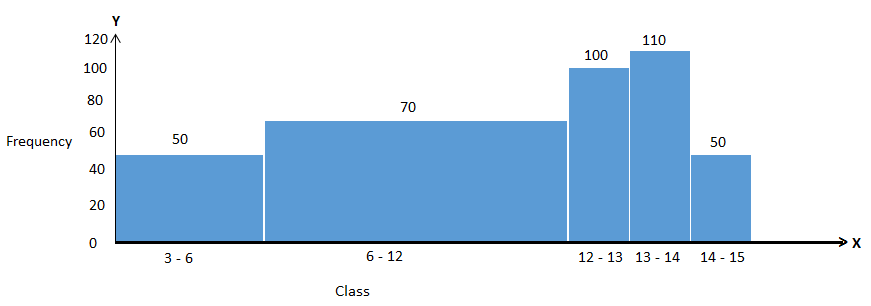Question 10: From the following table draw a histogram.

 Class 5 – 9 10 – 14 15 – 19 20 – 24 Frequency 3 5 8 2

Solution:

The given frequency distribution is grouped but not continuous. So, first we will have to convert it as regular.

Lower limit of 2nd class interval =10

Upper limit of 1 st class interval = 9

Difference (h) = 10 – 9 = 1

Therefore, h/2 = 1/2 = 0.5

By subtracting 0.5 from the lower limit and adding 0.5 to the upper limit we can make class intervals regular.

 Class 4.5 – 9.5 9.5 – 14.5 14.5 – 19.5 19.5 – 24.5 Frequency 3 5 8 2

Take class intervals on the X-axis and frequency values on the Y-axis with suitable scale.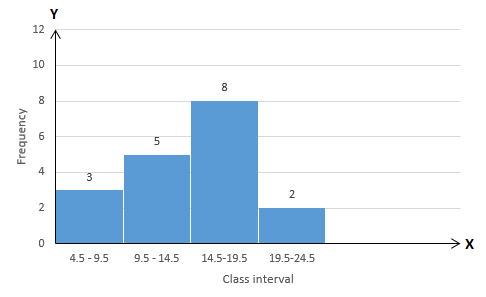Question 11: Draw a frequency polygon with the help of a histogram for the following distribution. Maximum number is 10.

 Marks 0 – 5 5 – 10 10 – 15 15 – 20 20 – 25 25 – 30 30 – 35 Frequency 1 2 4 6 5 3 2

Solution:

Take the marks on the X-axis and frequency values on the Y-axis.

After drawing the rectangles for the corresponding class intervals (i.e. histogram) mark the mid-points of each rectangle and join them with the lines as shown below.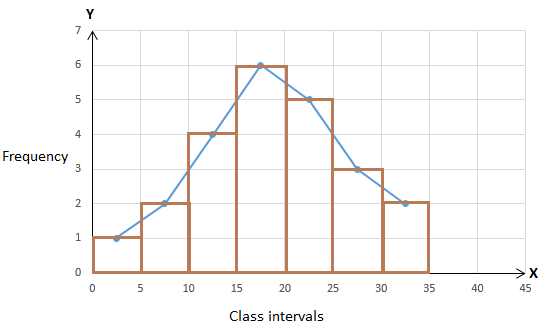Question 12: Draw a frequency polygon for the following frequency distribution.

 Variable (x) 5 10 15 20 25 30 Frequency (f) 2 6 4 1 5 2

Solution:

Take variable x on the X-axis and frequency values on the Y-axis.

Plot the following ordered pairs on a graph paper:

(5, 2), (10, 6), (15, 4), (20, 1), (25, 5), (30, 2)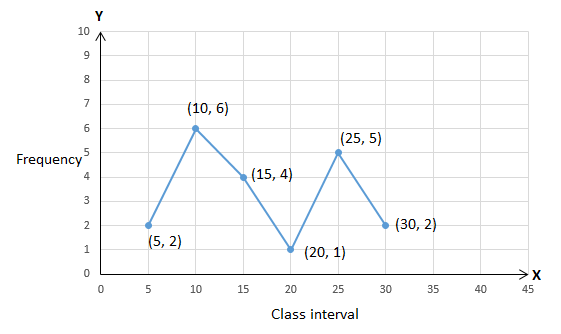Question 13: Draw a frequency polygon for the following frequency distribution.

 Production (Tonnes) 0 – 10 10 – 20 20 – 30 30 – 40 40 – 50 50 – 60 60 – 70 Frequency 8 18 23 37 47 26 16

Solution:

Let us find the class marks (mid-points) of the given class intervals.

 Production (Tonnes) Class mark (x) Frequency (f) Points 0 – 10 5 8 (5, 8) 10 – 20 15 18 (15, 18) 20 – 30 25 23 (25, 23) 30 – 40 35 37 (35, 37) 40 – 50 45 47 (45, 47) 50 – 60 55 26 (55, 26) 60 – 70 65 16 (65, 16)

Take the values of class marks on the X-axis and the frequency values on the Y-axis.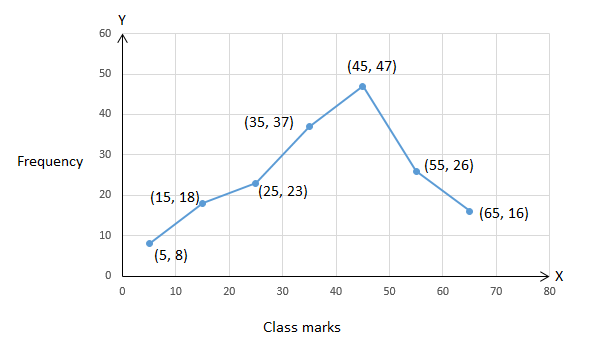## RBSE Maths Chapter 15: Exercise 15.4 Textbook Important Questions and Solutions

For more practice questions of the concepts mean, median and mode of the simple data students can visit here.

### RBSE Maths Chapter 15: Additional Important Questions and Solutions

Question 1: In a distribution 5, 5, 6, 4, 9, 5, 3, 2, 7, 6, 3, 8, 4: frequency of class interval 3 – 5 is

(A) 3

(B) 5

(C) 6

(D) 7

Solution:

Given,

5, 5, 6, 4, 9, 5, 3, 2, 7, 6, 3, 8, 4

For class interval 3-5: data values which are less than 5 will be included,i.e. 2, 3, 3, 4, 4

Therefore, frequency of the class interval 3 – 5 is 5.

Question 2: Range of the following distribution will be

3.2 2.8 3.1 2.1 3.2 2.4 2.1 2.8 2.7 2.7

(A) 2.7

(B) 3.1

(C) 2.4

(D) 1.1

Solution:

Given,

3.2 2.8 3.1 2.1 3.2 2.4 2.1 2.8 2.7 2.7

Maximum value = 3.2

Minimum value = 2.1

Range = Maximum value – Minimum value

= 3.2 – 2.1

= 1.1

Question 3: In the following distribution, number of students of age less than 25 years is

 Age (in years) 5 – 10 10 – 15 15 – 20 20 – 25 25 – 30 Number of students 3 6 8 8 2

(A) 8

(B) 16

(C) 9

(D) 25

Solution:

We know that, in continuous distribution the upper limits are not included in the class interval rather than included in the next class interval.

The number of students of age less than 25 years can be obtained by adding the number of students from the class interval 5 – 10 to 20 – 25.

Required number of students = 3 + 6 + 8 + 8 = 25

Question 4: In a bar graph, height of rectangle is

(A) inversely proportional to frequency of class

(B) proportional to frequency of class

(C) proportional to class-interval

(D) inversely proportional to class interval

Solution:

Histogram constructed according to the class intervals and the corresponding frequency. Here, height of the rectangle represents the frequency of class interval. Hence, height of the rectangle is inversely proportional to class interval.

Question 5: The examination result of any class of a school can be comparatively studied

(A) by circular diagram

(B) by bar graph

(C) by linear graph

(D) all of these

Solution:

Examination result of any class of a school contains two variables like marks and number of students. Here, marks can be taken as class intervals and the number of students can be considered as frequency. In this case, a bar graph is the best one to display the data and also it is to study and understand as well.

Question 6: The range of distribution 6, 1, 2, 3, 9, 8, 3, 4, 8, 2, 3 will be

(A) 4

(B) 8

(C) 7

(D) 6

Solution:

Given,

Given,

6, 1, 2, 3, 9, 8, 3, 4, 8, 2, 3

Maximum value = 9

Minimum value = 1

Range = Maximum value – Minimum value

= 9 – 1 = 8

Question 7: In the distribution 5, 1, 5, 2, 3, 6, 5, 4 frequency of 5 will be

(A) 1

(B) 2

(C) 3

(D) 4

Solution:

Given,

5, 1, 5, 2, 3, 6, 5, 4

Count the number of 5s:

5, 5, 5

Therefore, frequency of 5 is 3.

Question 8: The median of 11, 2, 7, 8, 9, 3, 5 will be

(A) 7

(B) 9

(C) 5

(D) 11

Solution:

Given,

11, 2, 7, 8, 9, 3, 5

Arrange the given data in ascending order:

2, 3, 5, 7, 8, 9, 11

Number of observations = 7 (odd)

Median = (7 +1)/2 th observation = 8/2 = 4th observation

Therefore, median = 7

Question 9: The mean of 15, 0, 10, 5 will be

(A) 15

(B) 10

(C) 5

(D) 7.5

Solution:

Given,

15, 0, 10, 5

Sum of observations = 15 + 0 + 10 + 5 = 30

Number of observations = 4

Mean = Sum of observations/ Number of observations

= 30/4

= 7.5

Question 10: In 4, 3, 4, 5, 4, 2, 4, 1, mode will be

(A) 1

(B) 2

(C) 5

(D) 4

Solution:

Given,

4, 3, 4, 5, 4, 2, 4, 1

Count the number of times the observation is repeated.

 Observation Frequency 1 1 2 1 3 1 4 4 5 1

Highest frequency is 4 for the observation 4.

Therefore, mode = 4

Question 11: If 7 is the mean of the numbers 5, 8, 4, x, 6, 9, then find the value of x.

Solution:

Given,

5, 8, 4, x, 6, 9

Sum = 5 + 8 + 4 + x + 6 + 9 = 32 + x

Number of observations = 6

Mean = Sum of observations/ Number of observations

7 = 32 + x/ 6

42 = 32 + x

x = 42 – 32 = 10

Question 12: What is range?

Solution:

The difference between the maximum and minimum value of the variable in the given data is called range.

Formula to find the range of the data is:

Range = Maximum value – Minimum value

Question 13: What is histogram?

Solution:

Histogram can be defined as the rectangular representation of classified (grouped) and continuous frequency distribution.

Question 14: Make a frequency table of 9, 7, 9, 8, 3, 9, 8, 3, 5, 7, 5, 3

Solution:

The frequency distribution table for the given data is:

 Variable (x) Tally Marks Frequency (f) 3 ||| 3 5 || 2 7 || 2 8 || 2 9 ||| 3

Question 15: If the arithmetic mean of a frequency distribution is 15 and ∑f = 20, then find the value of ∑fx.

Solution:

Given,

Arithmetic mean = 15

∑f = 20

We know that,

Arithmetic mean = ∑fx/∑f

15 = ∑fx/ 20

∑fx = 15 × 20 = 300

Therefore, ∑fx = 300

Question 16: Write median of distribution: 5, 2, 3, 7, 5, 4, 3, 2, 1

Solution:

Given,

5, 2, 3, 7, 5, 4, 3, 2, 1

Ascending order of the given observations:

1, 2, 2, 3, 3, 4, 5, 5, 7

Number of observations = n = 9 (odd)

Median = (n + 1)/2 th observation = (9 + 1)/2 = 10/2 = 5th observation

Therefore, median = 3

Question 17: Find median of distribution: 12, 1, 6, 4, 10, 8, 1, 4

Solution:

Given,

12, 1, 6, 4, 10, 8, 1, 4

Ascending order of the given observations:

1, 1, 4, 4, 6, 8, 10, 12

Number of observations = 8 (even)

Median = mean of (n/2) th and (n/2) + 1 th observations

= (4th + 5th observations)/2

= (4 + 6)/2

= 10/2

= 5

Therefore, median = 5

Question 18: Find mode of distribution 4, 3, 4, 1, 2, 4, 7, 5, 3

Solution:

Let us write the observations with their corresponding frequencies as shown below.

 Observation Frequency 1 1 2 1 3 2 4 3 5 1 7 1

Highest frequency is 3 which represents the observation 4.

Hence, mode of the given data is 4.

Question 19: Draw a frequency polygon with the help of histograms for the following frequency distribution:

 Marks 2 – 4 4 – 6 6 – 8 8 – 10 10 – 12 Number of students 7 8 4 9 2

Solution:

Take the marks on the X-axis and frequency values on the Y-axis.

After drawing the rectangles for the corresponding class intervals (i.e. histogram) mark the mid-points of each rectangle and join them with the lines as shown below.